### Knowing Address Function And Different Ways To Use It In Excel

In this lesson you can learn how to use ADDRESS function. By using the ADDRESS function, you can get the address of the cell in the spreadsheet, giving a specific row and column number.

Address function creates a text based on the address of cell in the spreadsheet.

The syntax is as follows:

=ADDRESS (row_num; column_num, [abs_num], [A1], [sheet_text])

• row_num and column_num are arguments which specify the location of the cell. For example, to create a text cell reference C2 are, respectively, the number of type 2 and 3
• abs_num is a kind of appeal to create a function, given as a number between 1-4, as follows:
1. (default) means the absolute reference (\$A\$1),
2. row absolute, relative to the column (A\$1),
3. relative to the row, absolute for the column (\$A1),
4.  relative reference (A1).
• A1 is an optional argument allows us to choose the type of appeal.

If you leave the field blank or write in the TRUE or 1, the address is created in the style of A1,

if the argument will be set to false or 0 – R1C1 style address.

• sheet_text is an optional argument allows you to specify the sheet that contains the cell. You must write the name of the sheet in quotation marks.

### Find MAX value in range

You have values in A1:A5 range. You want to find the address of cell with the greatest value. Formula is: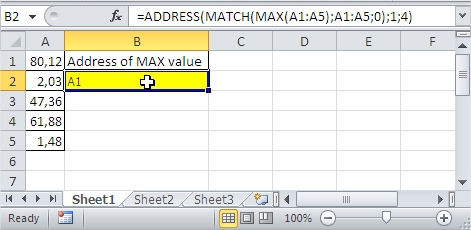### Find MIN value in range

You have values in A1:A5 range. You want to find the address of cell with the smallest value. Formula is: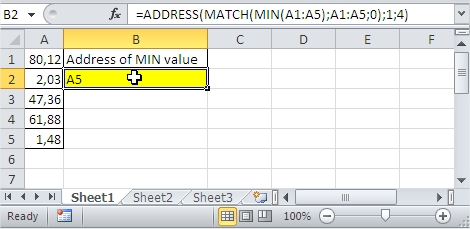### Simple Address Formula

This is under the circumstances that we are using the formula to find a specific address to numeric values that would make it possible to find the right value.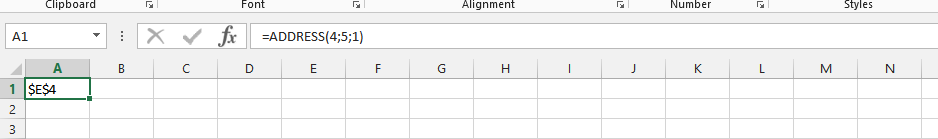### Address Formula with Text

This example is about specifying the address of a cell with formula that also has text. In this example, we are going to be using the address formula to get the address to this information.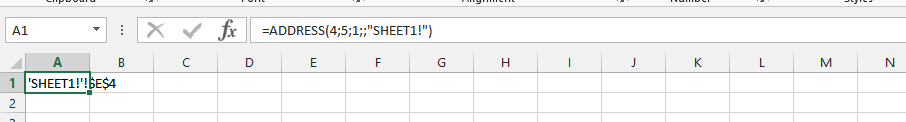### Address and Match Formula Simultaneously

In this example, we are using the match and address formula to find the address of the information given to the formula that has been written to it.### Address and Match in a Respective Formula

The example would be using both the match and address formula that would be used to find the answer.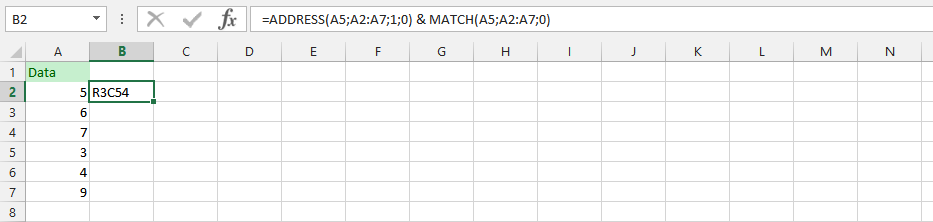### Match, Address and Max in One Formula

This example is combining the three formula into one single formula to find the address to one simple and yet comprehensive formula that makes finding the answer an easy process.### Three Different Formulas with Address

This example is about using the address, match and max formula, but this time it would be quite different, because there will be separation between the formulas.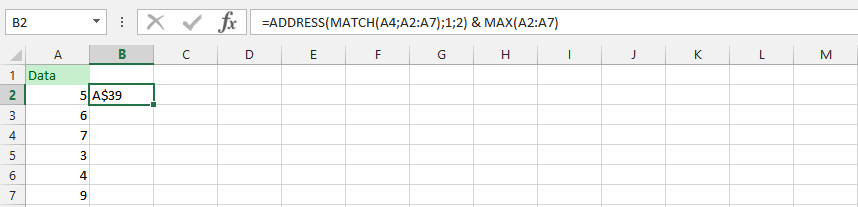### Formula for Finding Address with Min Formula

This formula is about finding the address, with two different formula that are in a single formula and get the answer that we are looking for, with the formula.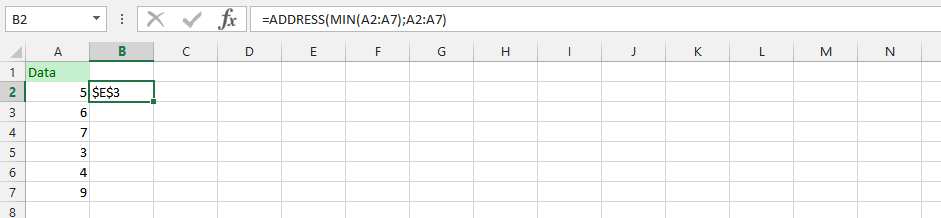### Min, Match and Address in a Single Formula

The formula is combining three different formulas to find the address for a specific information that we are looking for, and we are going to use the formula needed to get answers.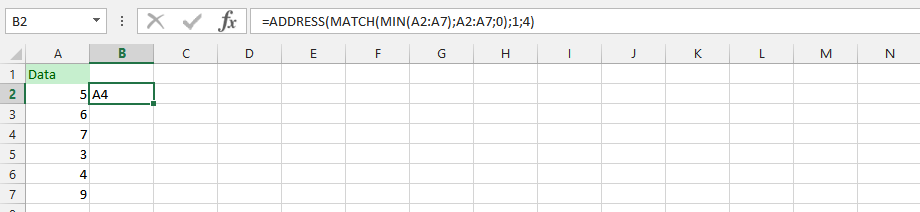### Using Three Different Formula in two Different Formulas

The example is focusing on finding the answer needed to perform a specific duty, and we need to know the address of the formulas.### Address and Min Formula with Different Spreadsheet

In this example, we’d have two different sheets, and wants to use another spreadsheet to find the address that we are looking for, with both the min and address formula.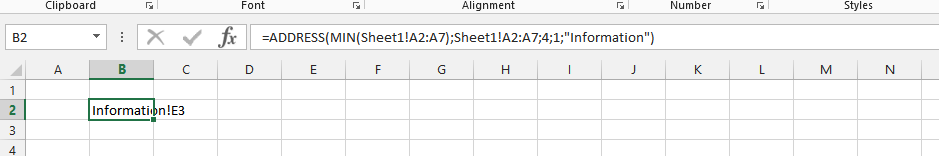## Template

```Further reading: